# 1. [25 points) Idealized frictionless free fall of an object that is dropped from being at...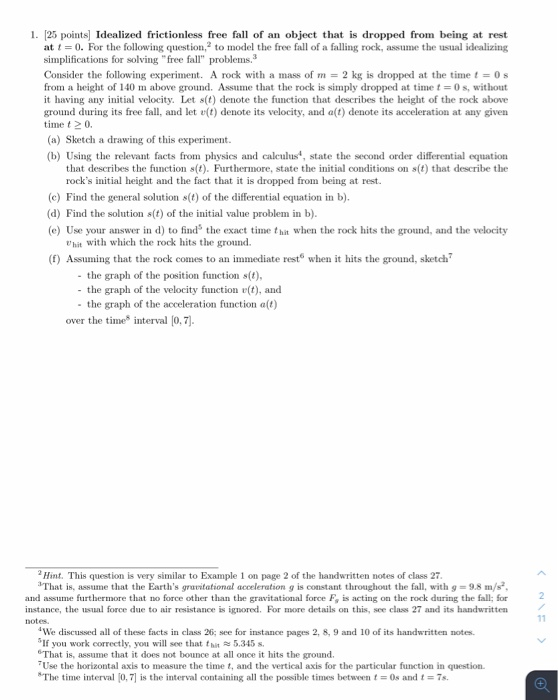1. [25 points) Idealized frictionless free fall of an object that is dropped from being at rest at i = 0. For the following question, to model the free fall of a falling rock, assume the usual idealizing simplifications for solving "free fall" problems. Consider the following experiment. A rock with a mass of m= 2 kg is dropped at the time t = 0 from a height of 140 m above ground. Assume that the rock is simply dropped at time t = 0 s, without it having any initial velocity. Let s(t) denote the function that describes the height of the rock above ground during its free fall, and let v(t) denote its velocity, and a(t) denote its acceleration at any given time to (a) Sketch a drawing of this experiment. (b) Using the relevant facts from physics and calculus, state the second order differential equation that describes the function s(t). Furthermore, state the initial conditions on s(t) that describe the rock's initial height and the fact that it is dropped from being at rest. (e) Find the general solution s(t) of the differential equation in b). (d) Find the solution s(t) of the initial value problem in b). (e) Use your answer in d) to find the exact time that when the rock hits the ground, and the velocity hit with which the rock hits the ground. (f) Assuming that the rock comes to an immediate rest when it hits the ground, sketch? - the graph of the position function s(t), - the graph of the velocity function r(t), and - the graph of the acceleration function alt) over the time interval (0.7). Hint. This question is very similar to Example 1 on page 2 of the handwritten notes of class 27. That is, assume that the Earth's gravitational acceleration g is constant throughout the fall, with g = 9.8 m/s, and assume furthermore that no force other than the gravitational force F, is acting on the rock during the fall; for instance, the usual force due to air resistance is ignored. For more details on this, see class 27 and its handwritten notes "We discussed all of these facts in class 26; see for instance pages 2, 8, 9 and 10 of its handwritten notes If you work correctly, you will see that th5.345 s. That is, assume that it does not bounce at all once it hits the ground. Use the horizontal axis to measure the time t, and the vertical axis for the particular function in question. The time interval (0.7) is the interval containing all the possible times between t = 0s and 1 = 7s.

Rate of change of velocity is acceleration, as in free falling of mass. Acceleration is gravitational acceleration that g = 9.8m/s^2

thus,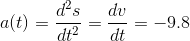m/s^2

acceleration is taken negative because it is acting downward.

integrating the differential equation: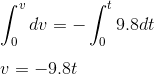m/s

Rate in change in displacement is velocity: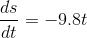Integrating this: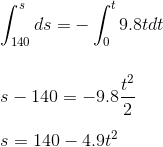(this is function of displacement from initial position with respect to time)

when rock hit the ground:

s = 0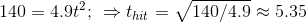second

velocity for the same: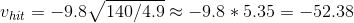m/s

(negative because direction of velocity is downward)

Position function graph. Impact at t = 5.35second. then velocity is zero shown till t = 7 second.

(quadratic function before impact), no motion after impact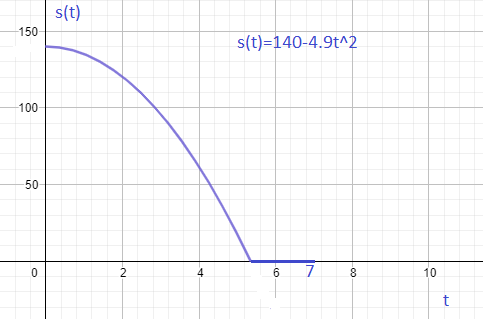Velocity function graph: (linear function)

Velocity become zero after impact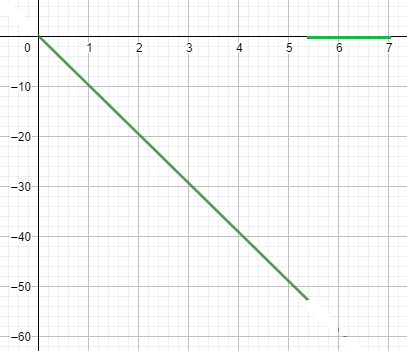Acceleration function graph (constant function)

Acceleration become zero after impact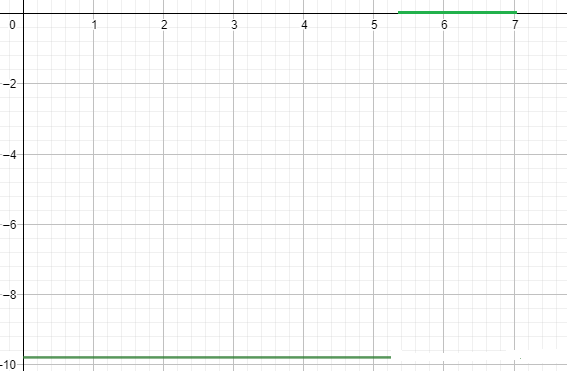#### Earn Coin

Coins can be redeemed for fabulous gifts.

Similar Homework Help Questions
• ### 6. [10 points) For the following question, to model the free fall of a falling rock,...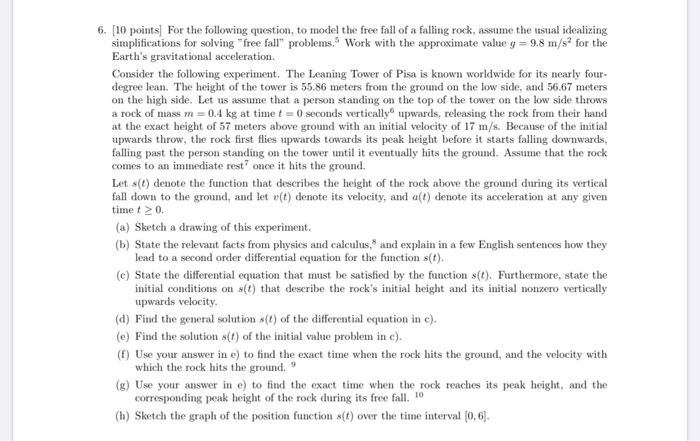6. [10 points) For the following question, to model the free fall of a falling rock, assume the usual idealizing simplifications for solving "free fall" problems. Work with the approximate value g = 9.8 m/s2 for the Earth's gravitational acceleration. Consider the following experiment. The Leaning Tower of Pisa is known worldwide for its nearly four- degree lean. The height of the tower is 55.86 meters from the ground on the low side, and 56.67 meters on the high side....

• ### 6. The tower of Pisa is 54.5 m tall. Assuming that Galileo dropped his object from...

6. The tower of Pisa is 54.5 m tall. Assuming that Galileo dropped his object from rest from the top of the tower and that the effects of air resistance were negligible, how long would it take the object to fall, in seconds? What is the velocity of the object when it hits the ground? 7. A ball is thrown directly downward with an initial speed of 9.50 m/s from a height of 30.0 m. After what time interval does...

• ### In this problem, you will apply kinematic equations to a jumping flea.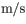problem 1) In this problem, you will apply kinematic equations to a jumping flea. Take the magnitude of free-fall acceleration to be 9.80m/s2 . Ignore air resistance.1. A flea jumps straight up to a maximum height of 0.510m. What is its initial velocity as it leaves the ground?3.16m/s2. How long is the flea in the air from the time it jumps to the time it hits the ground?time in air=____sProblem 2) A rock is thrown vertically upward with a speed of 19.0...

• ### QUIZ: Free fall and air drag Due Menday-Tuesday no exceptions Two objects are dropped simultaneously from...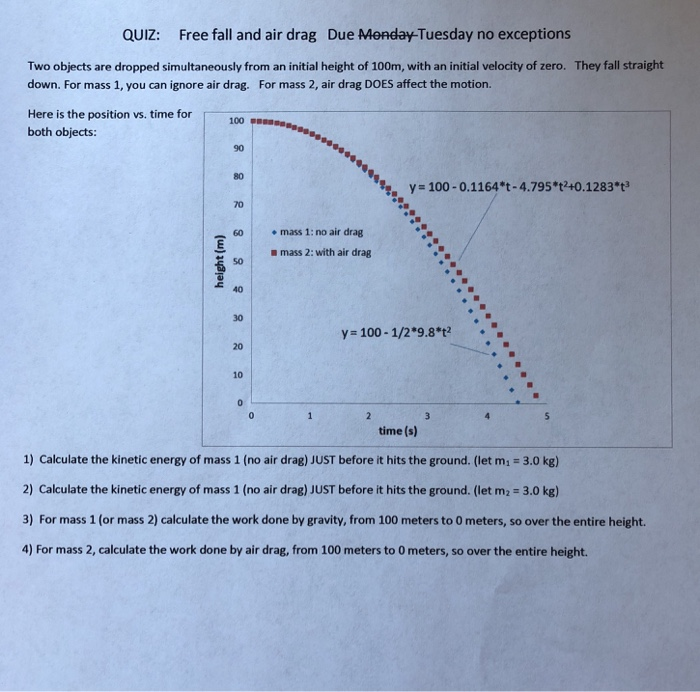QUIZ: Free fall and air drag Due Menday-Tuesday no exceptions Two objects are dropped simultaneously from an initial height of 100m, with an initial velocity of zero. They fall straight down. For mass 1, you can ignore air drag. For mass 2, air drag DOES affect the motion. Here is the position vs. time for both objects: 100 90 80 %, y-100-0.1164*t-4.795%40.1283*t3 70 60 mass 1: no air drag n mass 2: with air drag 50 40 30 y 100-1/2...

• ### 1:42 PM Wed Jun 5 79% Spring 2018 Final Exam Exam 3 Practice_a... X Spring 2018 Final E... Calculus Notes calculus home...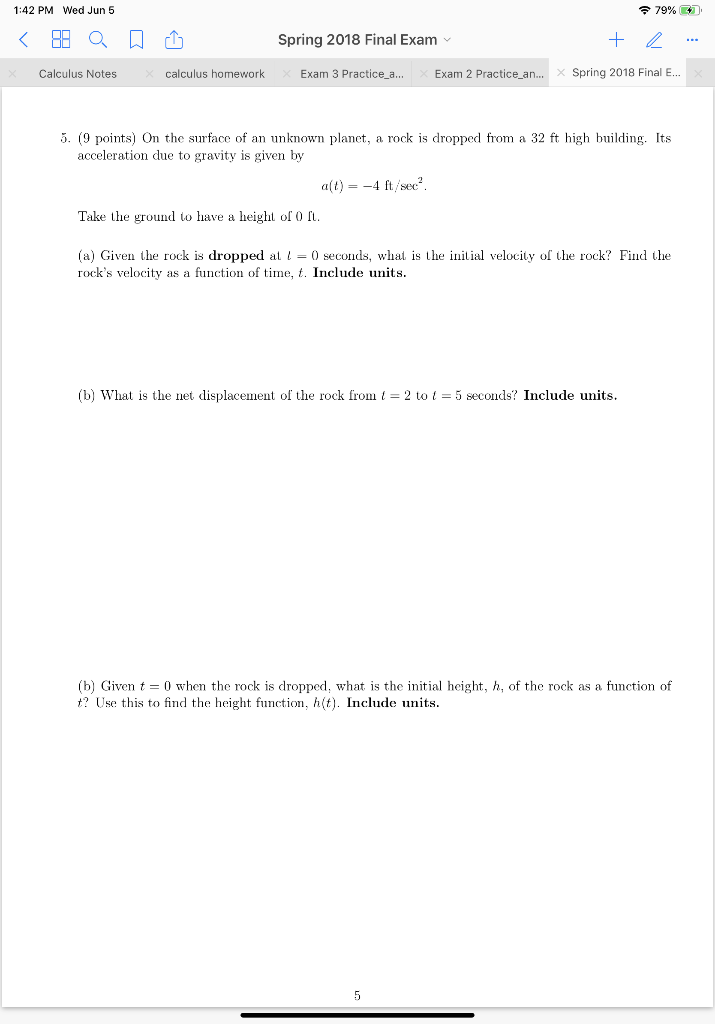1:42 PM Wed Jun 5 79% Spring 2018 Final Exam Exam 3 Practice_a... X Spring 2018 Final E... Calculus Notes calculus homework Exam 2 Practice_an... 5. (9 points) On the surface of an unknown planet, a rock is dropped from a 32 ft high building. Its acceleration due to gravity is given by a(t)-4 ft/sec Take the ground to have a height of 0 ft. (a) Given the rock is dropped at = 0 seconds, what is the initial velocity...

• ### 1. A car accelerates from zero to 105 km/h in 5.21 s. What is its average...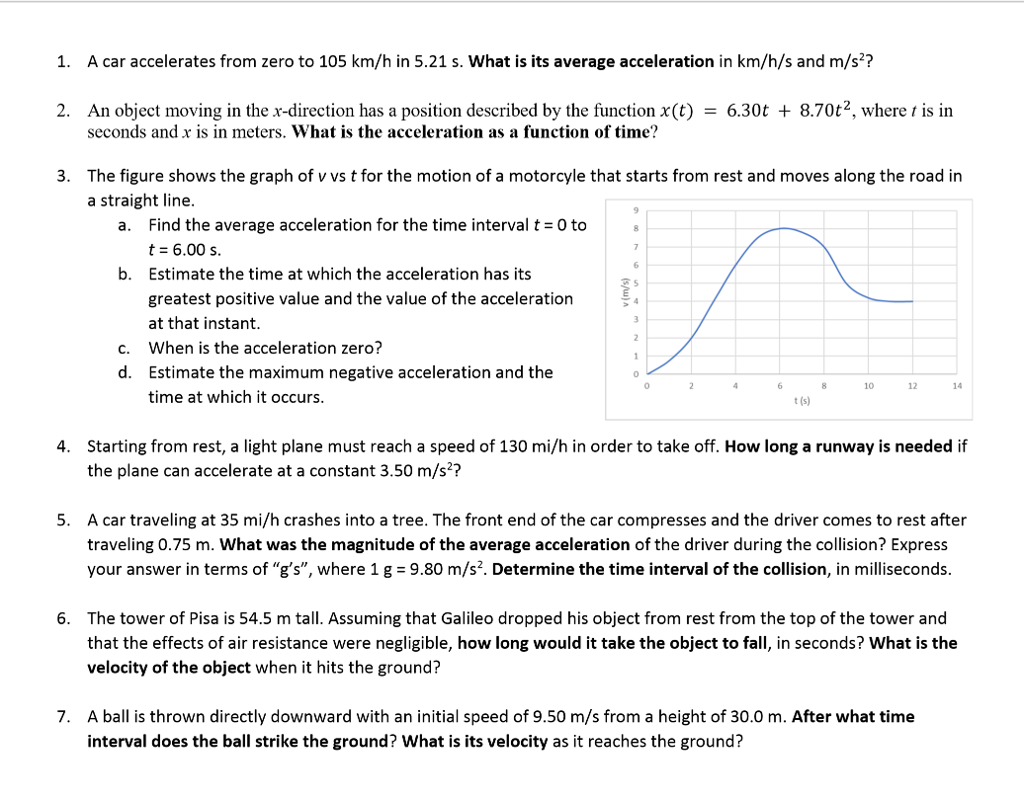1. A car accelerates from zero to 105 km/h in 5.21 s. What is its average acceleration in km/h/s and m/s? 2. An object moving in the x-direction has a position described by the function x(t) - 6.30t + 8.70t2, where t is in seconds and x is in meters. What is the acceleration as a function of time? The figure shows the graph of v vs t for the motion of a motorcyle that starts from rest and moves...

• ### An object moving in the *x direction experience an acceleration of *2.0 m/s^2- This mean the...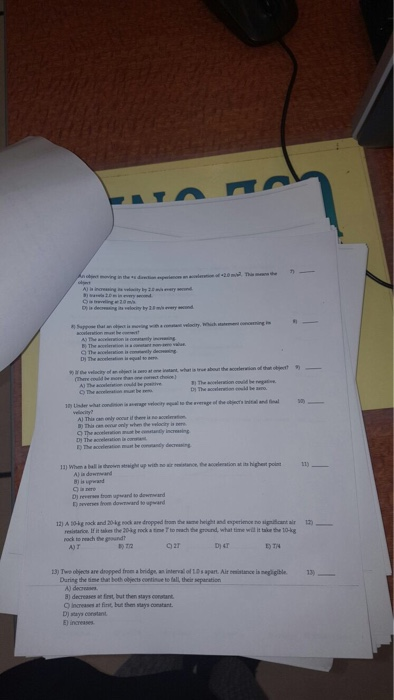An object moving in the *x direction experience an acceleration of *2.0 m/s^2- This mean the object is increasing its velocity by 2.0 m/s every second. travels 2.0 m In every second. is traveling at 2.0 m/s. is decreasing its velocity by 2.0 m/s every second. Suppose that an object is moving with a constant velocity. Which statement concerning Its acceleration must be correct? The acceleration is constantly increasing. The acceleration is a constant non-zero value. The acceleration is constantly...

• ### An object is dropped from a tower, 1024 ft above the ground. The object's height above...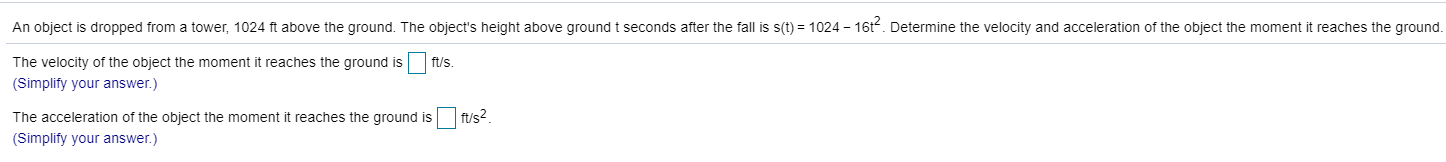An object is dropped from a tower, 1024 ft above the ground. The object's height above ground i seconds after the fall is s(t) = 1024 - 1612. Determine the velocity and acceleration of the object the moment it reaches the ground. ft/s. The velocity of the object the moment it reaches the ground is (Simplify your answer.) The acceleration of the object the moment it reaches the ground is fus2 (Simplify your answer.)

• ### Free Fall On the moon, the acceleration due to gravity is 1.6 m/sec2.(a) If a rock...

Free Fall On the moon, the acceleration due to gravity is 1.6 m/sec2.(a) If a rock is dropped into a crevasse, how fast will it be going just before it hits bottom 30 sec later?(b) How far below the point of release is the bottom of the crevasse?(c) If instead of being released from rest, the rock is thrown into the crevasse from the same point with a downward velocity of 4 m/sec, when will it hit the bottom and...

• ### A small object begins a free-fall from a height of H 865 m at to 0...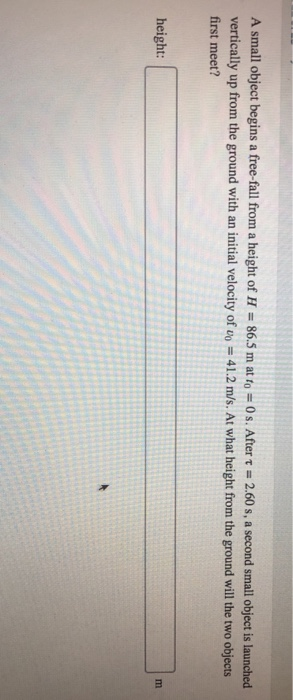A small object begins a free-fall from a height of H 865 m at to 0 s. After τ-2.60 s, a second small object is launched vertically up from the ground with an initial velocity of vo-41.2 m/s. At what height from the ground will the two objects first meet? height:

Free Homework Help App